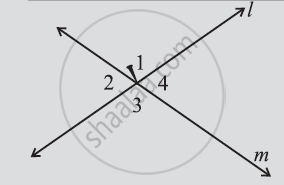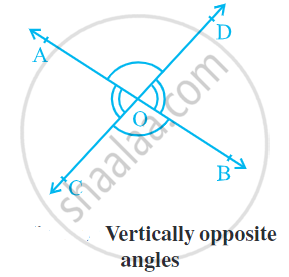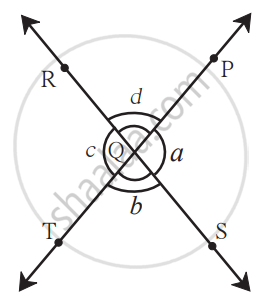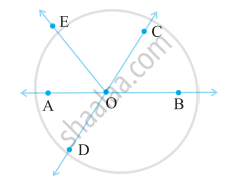# Related Angles - Concept of Vertically Opposite Angles

## Definition

Vertically Opposite Angles: When two lines intersect we have two pairs of opposite angles. They are called vertically opposite angles.

Point of intersection: The meeting point of two lines is called the point of intersection.

## Notes

### Vertically Opposite Angles:

• When two lines intersect we have two pairs of opposite angles. They are called vertically opposite angles.

• When two lines l and m meet, we say they intersect; the meeting point is called the point of intersection.

•• If two lines intersect each other, then the vertically opposite angles are equal.

• If two lines intersect at a point, and if one pair of vertically opposite angles are acute angles, then the other pair of vertically opposite angles are Obtuse angles.• Adjacent angles are linear and Supplementary angles i.e.,
∠AOC + ∠AOD = 180°.
∠AOD + ∠DOB = 180°.
∠DOB + ∠BOC = 180°.
∠BOC + ∠AOC = 180°.

## Theorem

### When two lines intersect, the vertically opposite angles so formed are equal.To Prove: ∠PQR and ∠SQT are congruent angles.

Proof:
As shown in the figure, m∠PQS = a, m∠SQT = b, m∠TQR = c, m∠PQR = d.
∠PQS and ∠SQT are the angles in a linear pair.
∴ a + b = 180°

Also, m∠SQT and m∠TQR are two angles in a linear pair.
∴ b + c = 180°
∴ a + b = b + c
∴ a = c.........(Subtracting b from both sides.)
∴ ∠PQS and ∠TQR are congruent angles.

Also, m∠PQR = m∠SQT
i.e. ∠PQR and ∠SQT are congruent angles.

Hence Proved.

## Example

In the given figure, Identify two pairs of vertically opposite angles.Vertically opposite angles are: (∠COB, ∠AOD), and (∠AOC, ∠BOD)

If you would like to contribute notes or other learning material, please submit them using the button below.

### Shaalaa.com

What are Vertically Opposite Angles? [00:09:21]
S
0%